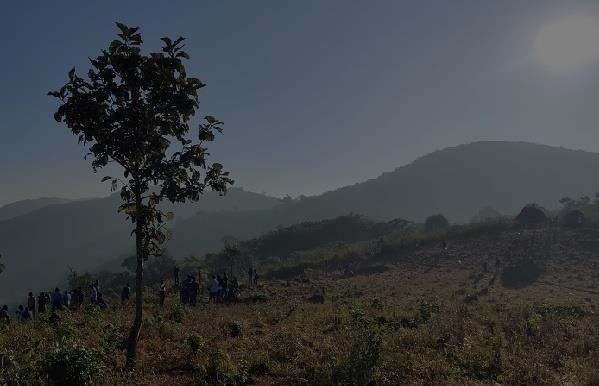# How to alter the contrast of an image using Java OpenCV library?

The increasing/decreasing of brightness and contrast of an image are the operations that can be achieved by transforming the pixels of the image. this can be expressed in the form of an equation as −

g(i, j) = α . f(i, j)+ β

Where,

• (i, j) are the positions of the pixels.

• α (gain) and β (bias) are the parameters of the transformation.

• At times the gain parameter controls the contrast of an image and the bias parameter controls the brightness of an image.

The convertTo() method of the org.opencv.core.Mat class performs the required calculations on the given matrix to alter the contrast and brightness of an image. This method accepts 4 parameters −

• mat − Empty matrix to hold the result with the same size and type as the source matrix.

• rtype − integer value specifying the type of the output matrix. If this value is negative, the type will be the same as the source.

• alpha − Gain value, which must be greater than 0 (default value 1).

• beta − Bias value (default value 0).

## Altering the contrast of an image using OpenCV Java library

As mentioned the alpha value passed to this method alters the contrast of an image, if the chosen value for this parameter is less than 1 (to 0) the contrast of the image is reduced. Similarly, if it is greater than 1 (to 255) the contrast of the image is increased.

To alter the contrast of an image −

• Read the contents of the desired image to a Mat object using the imread() method.

• Create an empty matrix with the same size and type as the matrix obtained in the previous step.

• Invoke the convertTo() method by passing the empty matrix, -1 (to get the same type), alpha value to increase or decrease contrast (0-1 or, 1-100) and, 0 as beta value.

• Write the contents of the resultant matrix as an output image using the Imgcodecs.imwrite() method.

## Example

import org.opencv.core.Core;
import org.opencv.core.Mat;
import org.opencv.imgcodecs.Imgcodecs;
public class AlteringContrast {
public static void main (String[] args) {
//Reading the Image from the file
String file ="D:\Images\car3.jpg";
//Creating an empty matrix
Mat dest = new Mat(src.rows(), src.cols(), src.type());
//Increasing the contrast of the image
src.convertTo(dest, -1, 10, 0);
// Writing the image
Imgcodecs.imwrite("D:\Images\altering_contrast_10.jpg", dest);
}
}

## Input ImageFollowing are the various output images for different alpha values −

α-value: 0.5α-value: 0.8α-value: 1.5α-value: 2.0